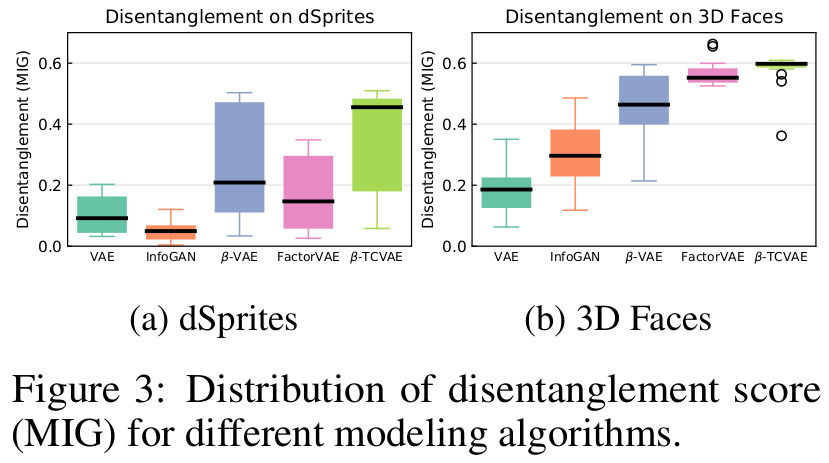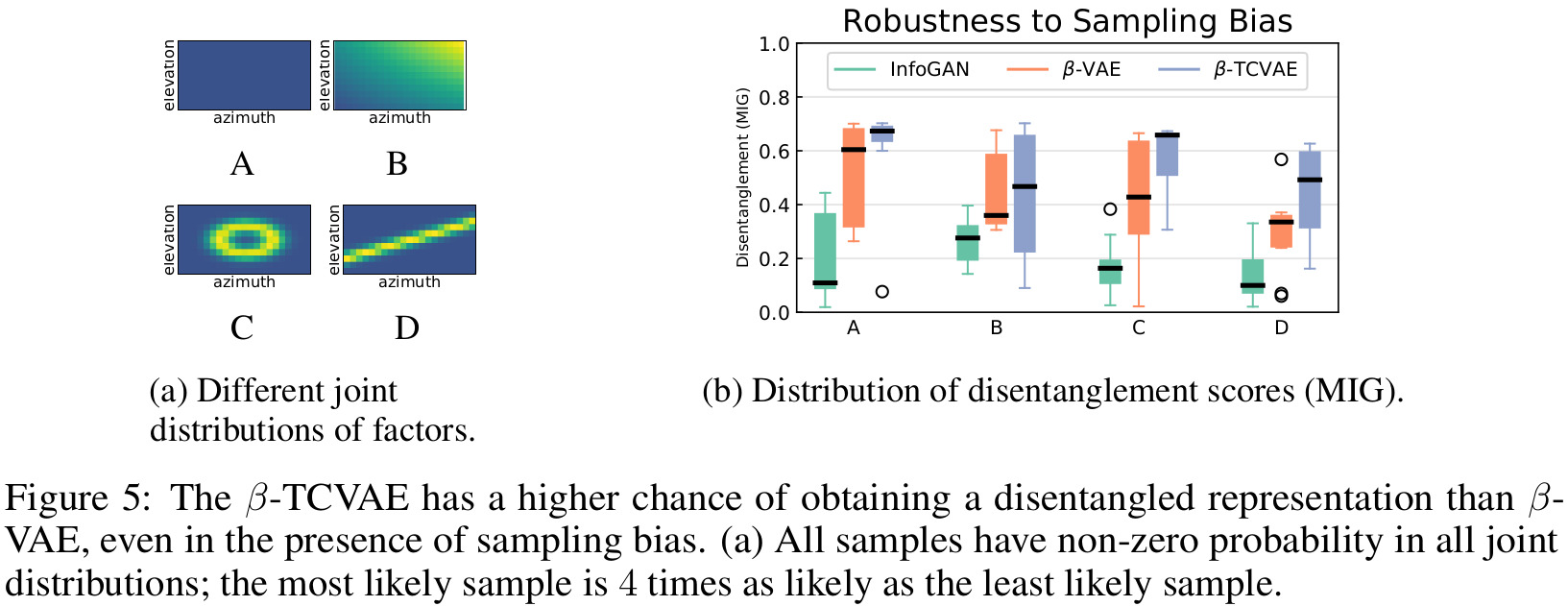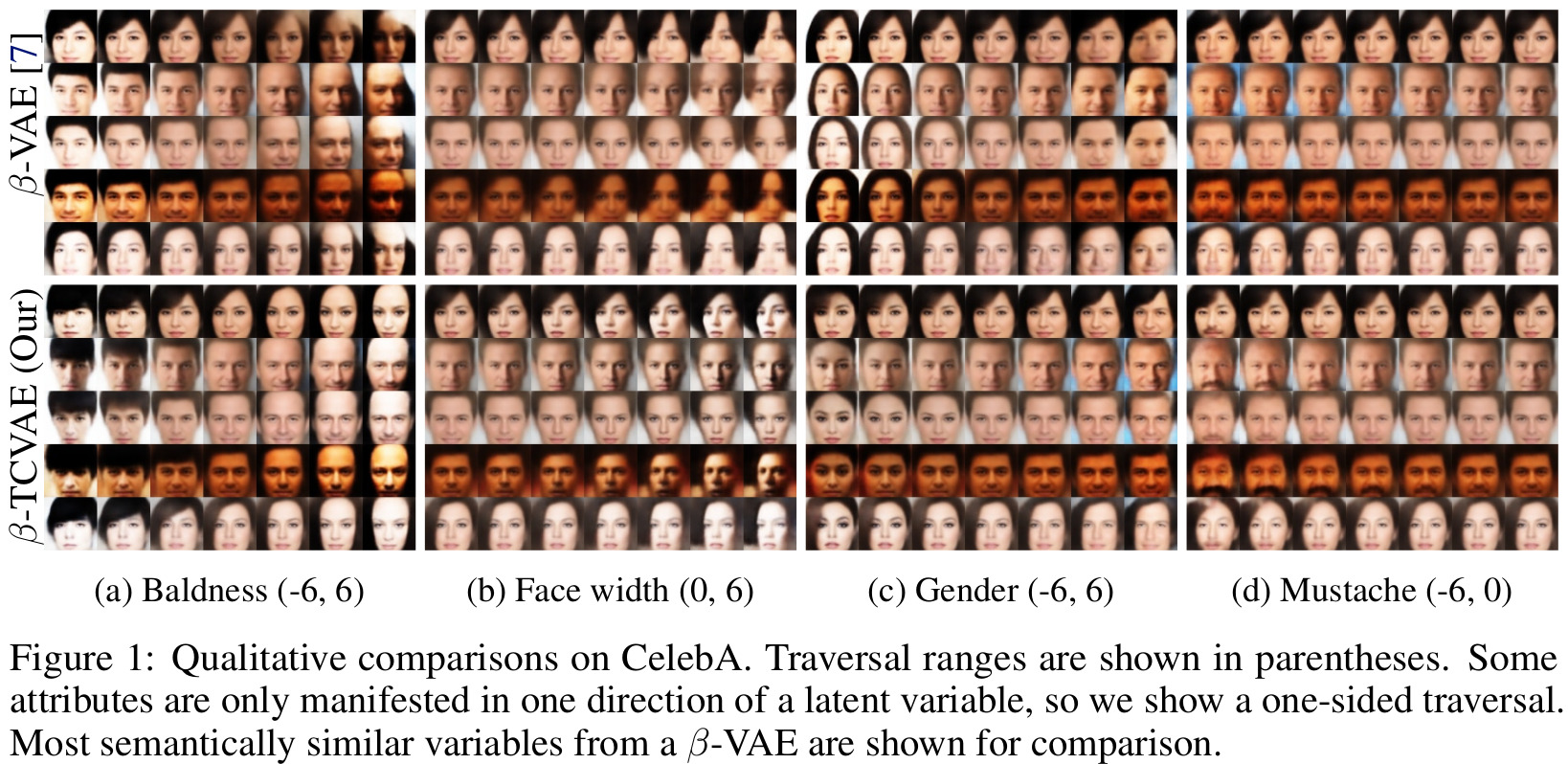# Highlights

• Decomposition of the variational lower bound that explains the success of $$\beta$$-VAE in learning disentangled representations
• Simple method based on weighted minibatches to estimate the aggregate posterior without additional hyperparameters (the aggregate posterior is required to weight specific terms in the decomposition of the ELBO)
• New $$\beta$$-TCVAE (Total Correlation Variational Autoencoder), which can be used as a plug-in replacement for the $$\beta$$-VAE (and VAE for that matter)
• New information-theoretic disentanglement metric, which is classifier-free and generalizable

# Introduction

The paper was written independently from a similar paper by Kim et Mnih1, and in the same vein propose a tweak on the $$\beta$$-VAE2 to further disentangle the learned representation without sacrificing the reconstruction, although the modifications proposed by both papers differ.

Let’s recall the objective of the $$\beta$$-VAE:

$\tag{1} \mathcal{L}_{\beta} = \frac{1}{N} \sum_{n=1}^{N} (\mathbb{E}_{q} [\log p(x_{n}|z)] - \beta KL(q(z|x_{n})~\|~p(z)))$

where $$\beta \gt 1$$ to encourage disentanglement. This is a more general formulation of the VAE, since we can obtain the original VAE objective by setting $$\beta = 1$$ in eq. 1.

# Methods

## ELBO TC-Decomposition

Let’s define $$q(z|n) = q(z|x_{n})$$ and $$q(z,n) = q(z|n) p(n) = q(z|n) \frac{1}{N}$$. The aggregate posterior is expressed as $$q(z) = \sum_{n=1}^{N} q(z|n) p(n)$$. The authors decompose the KL term like follows:

\begin{aligned} \tag{2} \mathbb{E}_{p(n)} [KL(q(z|n)~\|~p(z))] = & \underbrace{KL(q(z,n)~\|~q(z) p(n))}_{\text{1. Index-Code MI}} + \underbrace{KL(q(z)~\|~\prod_{j} q(z_{j}))}_{\text{2. Total Correlation}} \\ & + \underbrace{\sum_{j} KL(q(z_{j})~\|~p(z_{j}))}_{\text{3. Dimension-wise KL}} \end{aligned}

The authors describe the purpose of each term:

1. The index-code mutual information (MI) is the mutual information $$I_{q}(z;n)$$ between the data variable and latent variable;
2. The total correlation (TC) is a generalization of mutual information to more than two random variables. A heavier penalty on this term is what encourages a disentangled representation;
3. The dimension-wise KL prevents individual latent dimensions from deviating too far from their priors.

## Training with Minibatch-Weighted Sampling

The decomposed expression from eq. 2 requires to evaluate the aggregate posterior, which depends on the entire dataset and should thus not be computed during training. This is where Kim and Mnih1 used the density-ratio trick with a discriminator. Here, the authors propose a weighted version of a naïve Monte Carlo approximation, which they argue doesn’t require hyperparameters or inner optimization loops, to estimate the aggregate posterior.

Let $$\{n_1, \dots, n_M\}$$ be a minibatch of samples, their estimator is:

$\mathbb{E}_{q(z)}[\log q(z)] \approx \frac{1}{M} \sum_{i=1}^{M} \Bigg[\log \frac{1}{N~M} \sum_{j=1}^{M} q(z(n_{i})|n_{j})\Bigg]$

where $$z(n_{i})$$ is a sample from $$q(z \vert n_{i})$$ .

## $$\beta$$-TCVAE

The proposed $$\beta$$-TCVAE is simply assigning different weights to each of the terms in the ELBO TC-Decomposition:

$\mathcal{L}_{\beta - TC} = \mathbb{E}_{q(z|n) p(n)} [\log p(n|z) - \alpha I_{q}(z;n) - \beta KL(q(z)~\|~\prod_{j} q(z_{j})) - \gamma \sum_{j} KL (q(z_{j})~\|~p(z_{j}))]$

Through an ablation study, the authors claim that $$\alpha$$ and $$\gamma$$ don’t matter much in the results (they were set as $$\alpha = \gamma = 1$$ in the end), and that most improvements come from optimizing for $$\beta$$ (although they mention this behavior might be specific for each data set).

## Mutual Information Gap (MIG)

In order to compare the disentanglement performance of different methods, the authors also propose a parameter-free metric, whereas competing metrics from Higgins et al2 and Kim and Mnih1 both use a classifier.

The authors first propose an empirical mutual information (noted $$I_{n}(z_{j};v_{k})$$) and detailed in the paper) that measures the mutual information between latent variables ($$z_{j}$$) and underlying factors of the generative process ($$v_{k}$$). Based on that measure, they present the full mutual information gap (MIG):

$\frac{1}{K} \sum_{k=1}^{K} \frac{1}{H(v_{k})} \Bigg(I_{n}(z_{j^{(k)}};v_{k}) - \max_{j \neq j^{(k)}} I_{n}(z_{j};v_{k})\Bigg)$

where $$j^{(k)} = \argmax_{j} I_{n}(z_{j};v_{k})$$ and $$K$$s are the factors of the generative process. The division by $$H(v_{k})$$ (bound of the empirical mutual information) ensures that MIG is bounded by 0 and 1.

# Data

Experiments were performed on two data sets with known generative factors (dSprites and 3D Faces) and one unsupervised data set (CelebA).

# Results1. Review of FactorVAE introduced in “Disentangling by Factorising”: https://vitalab.github.io/article/2020/08/13/DisentanglingByFactorising.html  2 3

2. $$\beta$$-VAE paper from ICLR 2017: https://openreview.net/pdf?id=Sy2fzU9gl  2